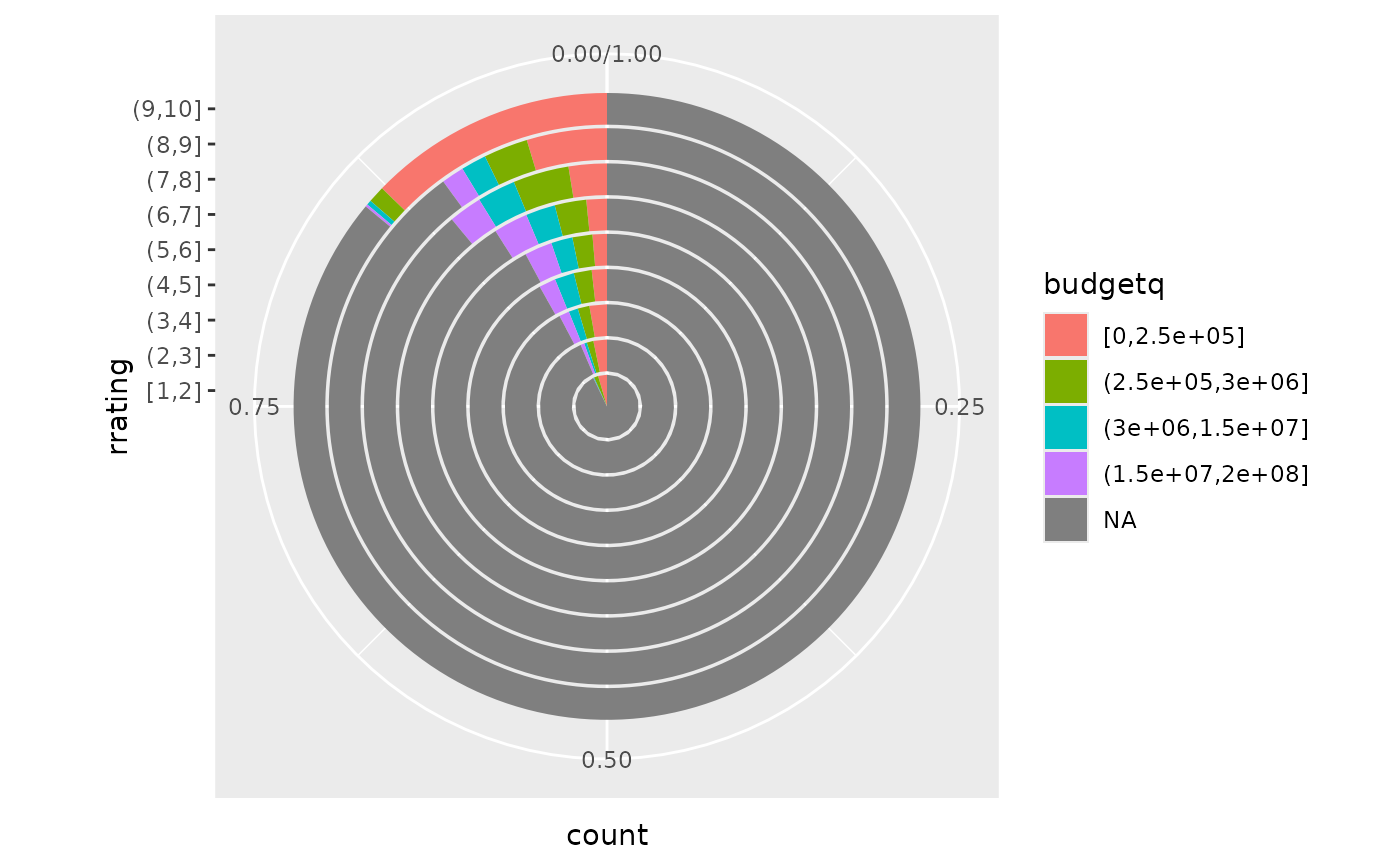The polar coordinate system is most commonly used for pie charts, which are a stacked bar chart in polar coordinates.

## Usage

coord_polar(theta = "x", start = 0, direction = 1, clip = "on")

## Arguments

theta

variable to map angle to (x or y)

start

Offset of starting point from 12 o'clock in radians. Offset is applied clockwise or anticlockwise depending on value of direction.

direction

1, clockwise; -1, anticlockwise

clip

Should drawing be clipped to the extent of the plot panel? A setting of "on" (the default) means yes, and a setting of "off" means no. For details, please see coord_cartesian().

## Examples

# NOTE: Use these plots with caution - polar coordinates has
# major perceptual problems.  The main point of these examples is
# to demonstrate how these common plots can be described in the
# grammar.  Use with EXTREME caution.

#' # A pie chart = stacked bar chart + polar coordinates
pie <- ggplot(mtcars, aes(x = factor(1), fill = factor(cyl))) +
geom_bar(width = 1)
pie + coord_polar(theta = "y")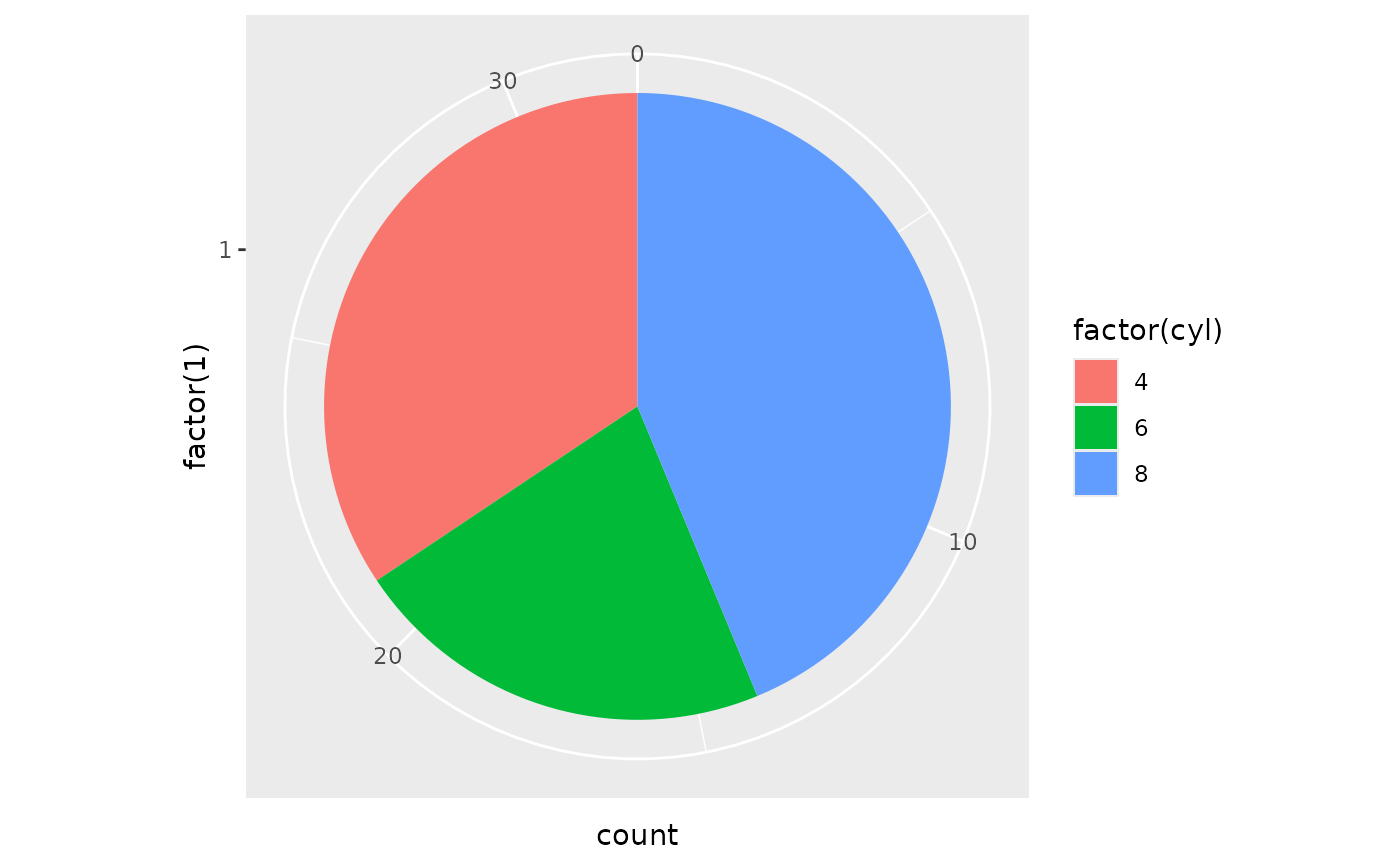# \donttest{

# A coxcomb plot = bar chart + polar coordinates
cxc <- ggplot(mtcars, aes(x = factor(cyl))) +
geom_bar(width = 1, colour = "black")
cxc + coord_polar()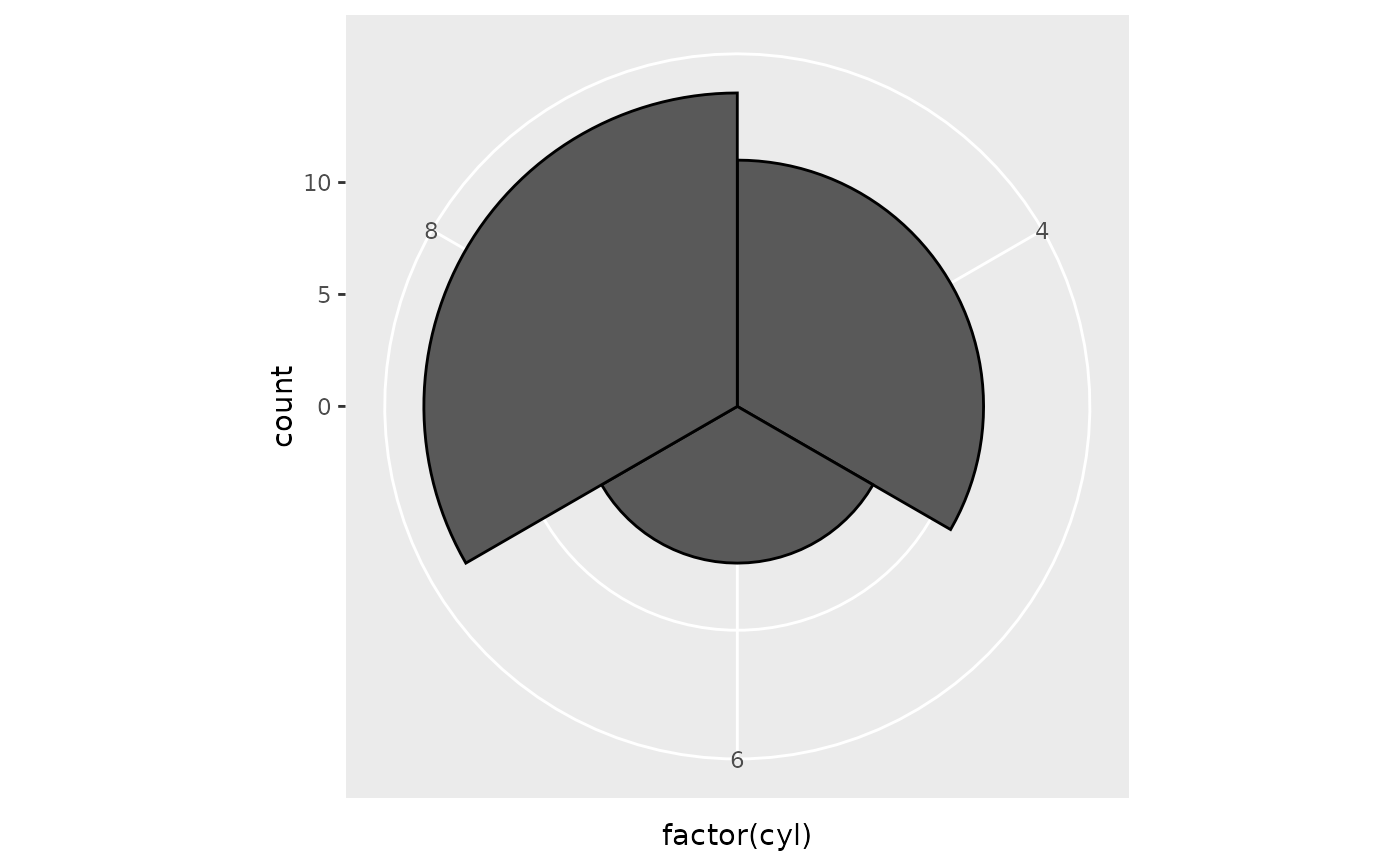# A new type of plot?
cxc + coord_polar(theta = "y")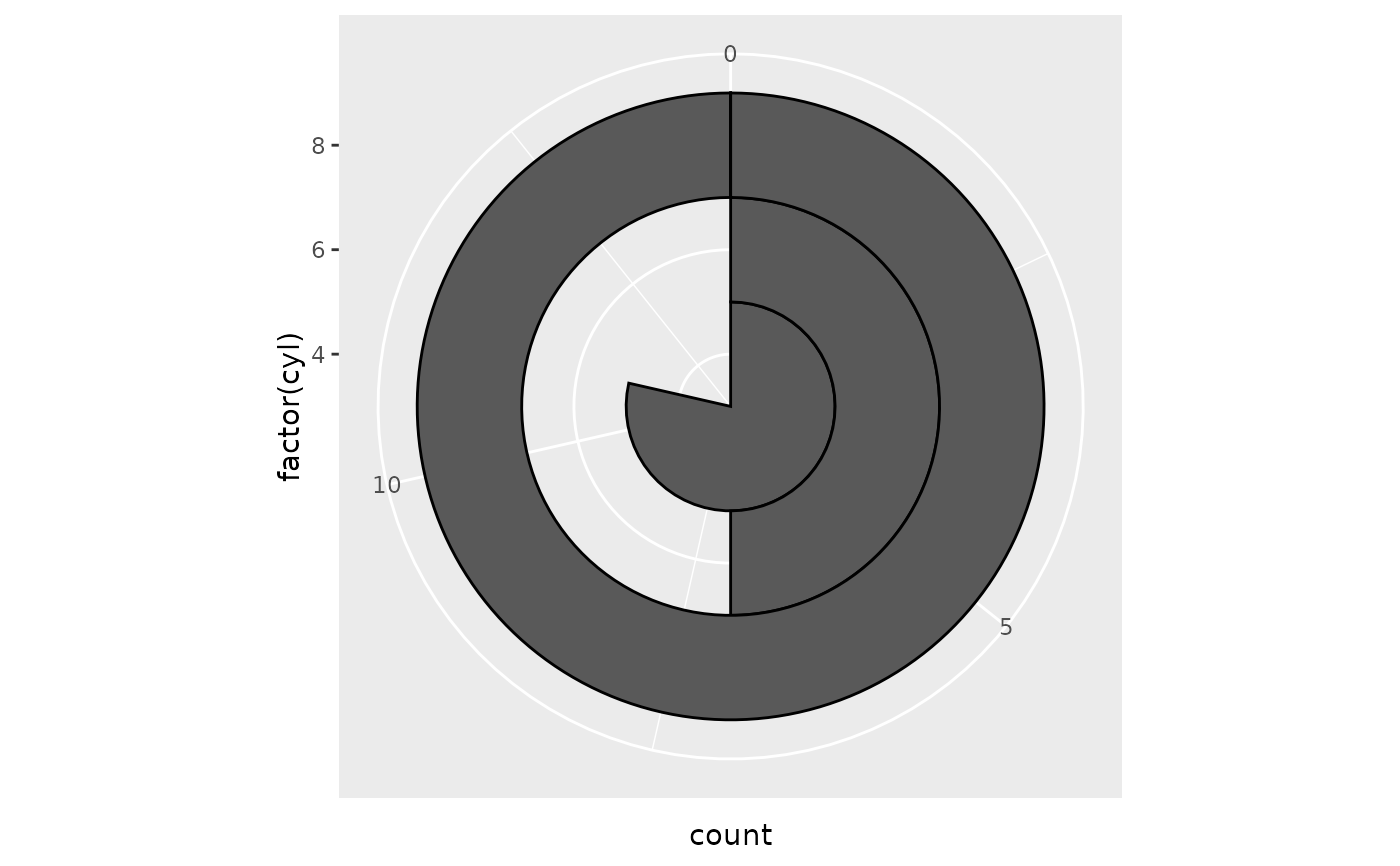# The bullseye chart
pie + coord_polar()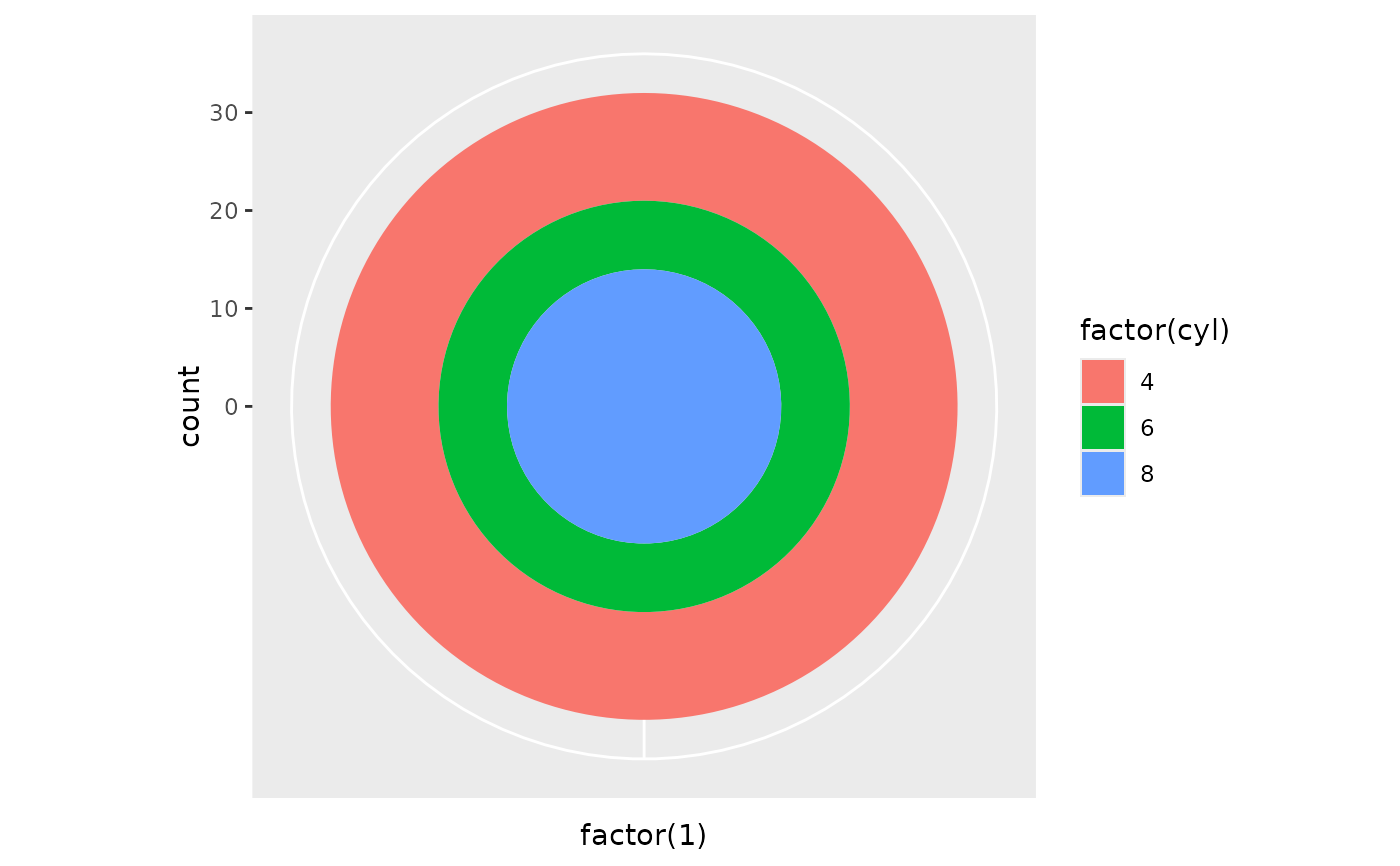df <- data.frame(
variable = c("does not resemble", "resembles"),
value = c(20, 80)
)
ggplot(df, aes(x = "", y = value, fill = variable)) +
geom_col(width = 1) +
scale_fill_manual(values = c("red", "yellow")) +
coord_polar("y", start = pi / 3) +
labs(title = "Pac man")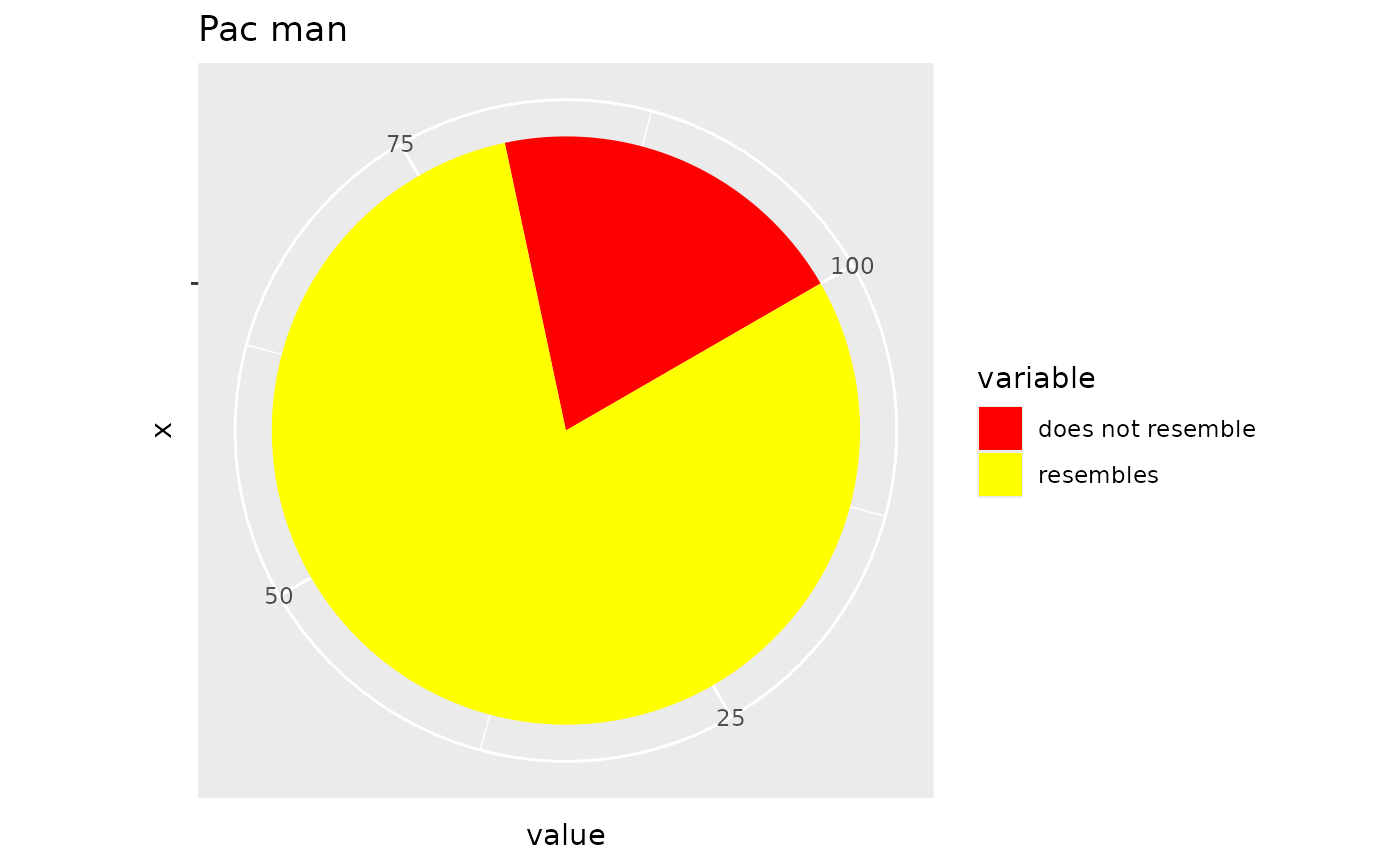# Windrose + doughnut plot
if (require("ggplot2movies")) {
movies$rrating <- cut_interval(movies$rating, length = 1)
movies$budgetq <- cut_number(movies$budget, 4)

doh <- ggplot(movies, aes(x = rrating, fill = budgetq))

# Wind rose
doh + geom_bar(width = 1) + coord_polar()
# Race track plot
doh + geom_bar(width = 0.9, position = "fill") + coord_polar(theta = "y")
}